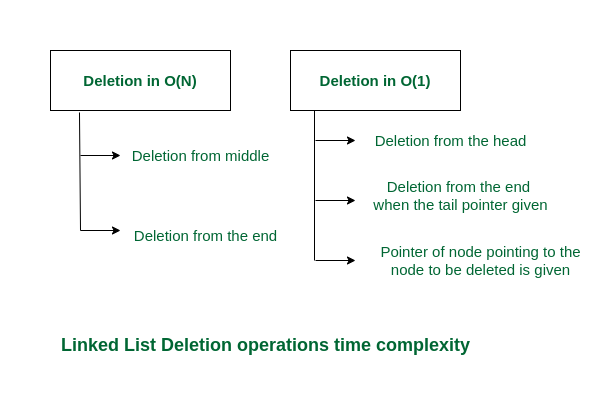Open in App
Not now

# Why is deleting in a Singly Linked List O(1)?

• Last Updated : 20 Dec, 2022

## How does deleting a node in Singly Linked List work?

To delete a node from a linked list we need to break the link between that node and the one pointing to that node. Assume node X needs to be deleted and node Y is the one pointing to X. So, we will need to break the link between X and Y and Y will point to the node which was being pointed by X.

## Types of Deletion of a node from Linked List

There can be three types of deletions:

1. Deletion from the beginning of the linked list
2. Deletion from the end of the linked list
3. Deletion from in between the beginning and the end.

All the cases do not take O(1) time. Check the below section to find the time complexities of different operations.

## Time Complexities of different deletion operations

Deletion from the beginning:

• Point head to the next node
• Time Complexity: O(1)

Deletion from the end:

• Traverse to the second last element
• Change its next pointer to NULL.
• Time Complexity: O(N)

#### Delete from the middle of a linked list

• Traverse to the element which is just before the element to be deleted
• Change the next pointers to exclude the node from the list
• Time Complexity: O(N)

## When Deletion in a Singly Linked List takes O(1) time?

There are 3 cases when deleting in a singly linked list is O(1), because we do not have to traverse the list.

First case: When we have the pointer pointing to the node which needs to be deleted, let’s call it prev. So, we have to do,

• curr = prev->next
• prev->next = curr->next
• curr->next = NULL
• delete curr

Since curr is the node that is deleted from the singly linked list.

Second case: When we have to delete the first/start/head node, let’s call it head. So, we have to do,

Hence head is pointing to the next node.

Third case: When we have to delete the last/end/tail node, let’s call it tail. So, we have to do,

• tail = NULL

This is only possible if we maintain an extra pointer except the head i.e., tail for referencing the last node of the linked list. But we can do this only once because after doing this we lose the reference of the last node and there is no way to find the reference of the new last node in O(1) in a singly linked list. In a doubly linked list, it is possible as it contains the previous pointer.Complexities of deletion from Linked List

Below is the implementation of different deletion operations in a singly linked list:

## C++

 `// C++ code to implement the approach`   `#include ` `using` `namespace` `std;`   `// Creating a class Node` `class` `Node {` `public``:` `    ``int` `data;` `    ``Node* next;` `    ``Node(``int` `d)` `    ``{` `        ``data = d;` `        ``next = NULL;` `    ``}` `};`   `// Function to delete the start node` `void` `deleteFromStart(Node*& head)` `{` `    ``head = head->next;` `}`   `// Function to delete the middle node` `void` `deleteGivenNode(Node*& head, ``int` `val)` `{` `    ``if` `(head->data == val) {` `        ``head = head->next;` `    ``}`   `    ``Node *temp = head, *prev = NULL;`   `    ``while` `(temp->data != val) {` `        ``prev = temp;` `        ``temp = temp->next;` `    ``}` `    ``prev->next = temp->next;` `}`   `// Function to delete the last node` `void` `deleteFromEnd(Node* head)` `{` `    ``Node* temp = head;`   `    ``while` `(temp->next->next != NULL) {` `        ``temp = temp->next;` `    ``}`   `    ``temp->next = NULL;` `}`   `// Function to print the list` `void` `printList(Node* head)` `{` `    ``Node* temp = head;` `    ``while` `(temp != NULL) {` `        ``cout << temp->data << ``" "``;` `        ``temp = temp->next;` `    ``}` `    ``cout << endl;` `}`   `// Driver Code` `int` `main()` `{` `    ``Node* head = ``new` `Node(10);` `    ``Node* second = ``new` `Node(20);` `    ``Node* third = ``new` `Node(30);` `    ``Node* fourth = ``new` `Node(40);`   `    ``head->next = second;` `    ``second->next = third;` `    ``third->next = fourth;`   `    ``cout << ``"Original list: "``;` `    ``printList(head);`   `    ``deleteFromStart(head);` `    ``cout << ``"List after deleting the starting node: "``;` `    ``printList(head);`   `    ``deleteFromEnd(head);` `    ``cout << ``"List after deleting the ending node: "``;` `    ``printList(head);`   `    ``deleteGivenNode(head, 30);` `    ``cout << ``"List after deleting the given node: "``;` `    ``printList(head);` `}`

## Python3

 `# Python code to implement the approach`   `# Creating a class Node` `class` `Node:` `    ``def` `__init__(``self``,val):` `        ``self``.val ``=` `val` `        ``self``.``next` `=` `None` `    `  `# Function to delete the start node` `def` `deleteFromStart(head):` `  ``if` `head !``=` `None``:` `    ``head ``=` `head.``next` `  ``return` `head` `  `  `#  Function to delete the middle node` `def` `deleteGivenNode(head,val):` `  ``if` `head.val ``=``=` `val: head ``=` `head.``next` `  ``curr ``=` `head` `  ``prev ``=` `None` `  ``while` `curr ``and` `curr.val !``=` `val:` `    ``prev ``=` `curr` `    ``curr ``=` `curr.``next` `  ``prev.``next` `=` `curr.``next` `  `  `# Function to delete the last node` `def` `deleteFromEnd(head):` `  ``curr ``=` `head` `  ``while` `curr.``next` `and` `curr.``next``.``next` `!``=` `None``:` `    ``curr ``=` `curr.``next` `  ``curr.``next` `=` `None`   `# Prints the stack` `def` `printList(head):` `  ``if` `head ``=``=` `None``:` `    ``print``(``'List is Empty'``)` `    ``return` `  ``curr ``=` `head` `  ``while` `curr !``=` `None``:` `    ``print``(curr.val,end ``=` `' '``)` `    ``curr ``=` `curr.``next` `  ``print``()`   `# Driver code` `head ``=`  `Node(``10``)` `second ``=` `Node(``20``)` `third ``=` `Node(``30``)` `fourth ``=` `Node(``40``)`   `head.``next` `=` `second ` `second.``next` `=` `third ` `third.``next` `=` `fourth `   `print``(``"Original list: "``)` `printList(head) `   `head ``=` `deleteFromStart(head) ` `print``(``"List after deleting the starting node: "` `)` `printList(head) `   `deleteFromEnd(head) ` `print``(``"List after deleting the ending node: "` `)` `printList(head) `   `deleteGivenNode(head, ``30``) ` `print``(``"List after deleting the given node: "``)` `printList(head)`

Output

```Original list: 10 20 30 40
List after deleting the starting node: 20 30 40
List after deleting the ending node: 20 30
List after deleting the given node: 20 ```

My Personal Notes arrow_drop_up
Related Articles